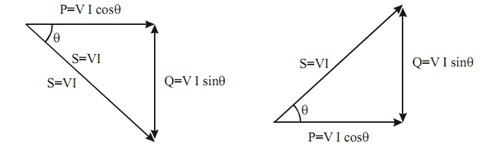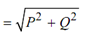## Power Triangle Assignment Help

Assignment Help: >> Power in AC Circuit - Power Triangle

Power Triangle:

All of the three power S, P and Q can be represented in a triangle, called the power triangle as illustrated in Figure.Figure : Power Triangle

Power may also be represented in complex form.

S = P + jQ

Magnitude  S  =